# GSEB Solutions Class 11 Statistics Chapter 4 Measures of Dispersion Ex 4.4

Gujarat Board Statistics Class 11 GSEB Solutions Chapter 4 Measures of Dispersion Ex 4.4 Textbook Exercise Questions and Answers.

## Gujarat Board Textbook Solutions Class 11 Statistics Chapter 4 Measures of Dispersion Ex 4.4

Question 1.
The marks obtained by 9 students in a test of 100 marks in Mathematics are given below:
64, 63, 72, 65, 68, 69, 66, 67, 69
Find the standard deviation of marks obtained by the students.
Here n = 9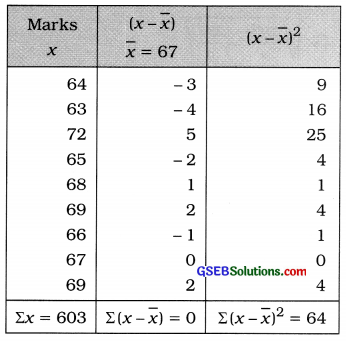Mean:
x̄ = $$\frac{\Sigma x}{n}=\frac{603}{9}$$ = 67 marks

Standard deviation of marks:
s = $$\sqrt{\frac{\Sigma(x-\bar{x})^{2}}{n}}$$
= $$\sqrt{\frac{64}{9}}$$
= $$\sqrt{7.111}$$
= 2.67 marksQuestion 2.
The numbers of cars coming for service in five service stations of a company on a particular day are 7, 3, 11, 8, 9. Calculate the standard deviation of number of cars coming at the service station.
Here, n = 5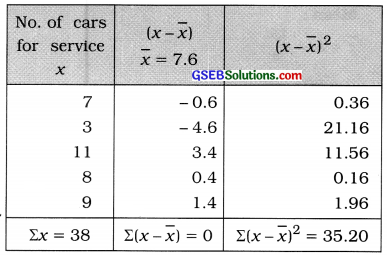Mean:
x̄ = $$\frac{\Sigma x}{n}$$
= $$\frac{38}{5}$$
= 7.6 Cars

Standard deviation of number of cars for service:
S = $$\sqrt{\frac{\Sigma(x-\bar{x})^{2}}{n}}$$
= $$\sqrt{\frac{35.20}{5}}$$
= $$\sqrt{7.04}$$
= 2.65 cars

Question 3.
The following frequency distribution represents the amounts of deposits and the number of depositors in a bank. Find the coefficient of standard deviation of the deposits.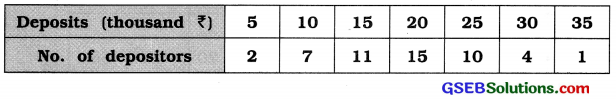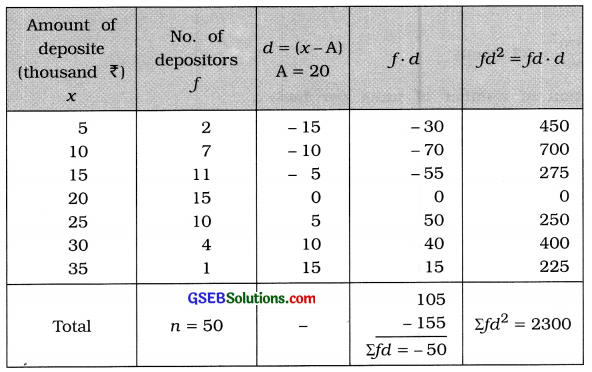Mean:
x̄ = A + $$\frac{\Sigma f d}{n}$$
= 20 – $$\frac{50}{50}$$
= 20 – 1
= ₹ 19 thousand

Standard deviation of amount of deposites:
s = $$\sqrt{\frac{\Sigma f d^{2}}{n}-\left(\frac{\Sigma f d}{n}\right)^{2}}$$
= $$\sqrt{\frac{2300}{50}-\left(\frac{-50}{50}\right)^{2}}$$
= $$\sqrt{46-(-1)^{2}}$$
= $$\sqrt{46-1}=\sqrt{45}$$ = ₹ 6.71 thousand

Coefficient of standard deviation
= $$\frac{s}{\bar{x}}=\frac{6.71}{19}$$ = 0.35Question 4.
The information of profits (in lakh ?) of 50 firms in the last year is given below. Find the standard deviation of the profit of the firms.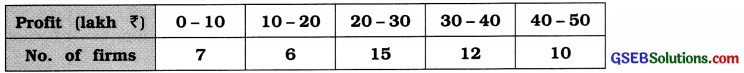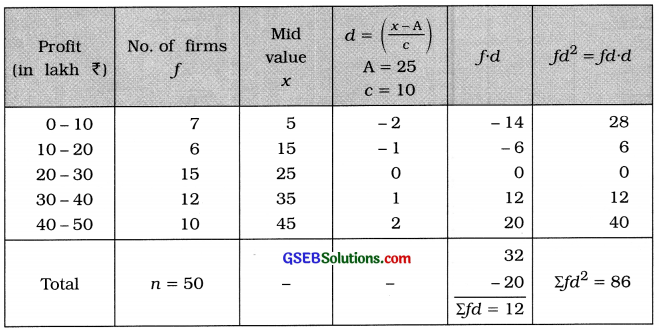Mean:
x̄ = A + $$\frac{\Sigma f d}{n}$$ × c
= 25 + $$\frac{12}{50}$$ × 10
= 25 + $$\frac{12}{5}$$
= 25 + 2.4
= ₹ 27.4 lakh

Standard deviation of profit: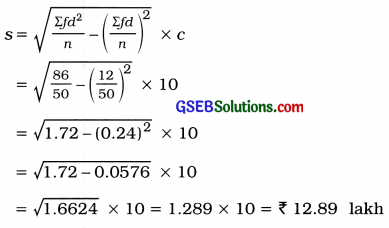Question 5.
Find the standard deviation of age of the persons from the following distribution of 125 persons living in a society. Also find the coefficient of standard deviation.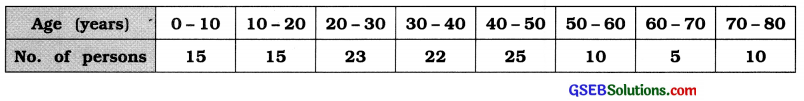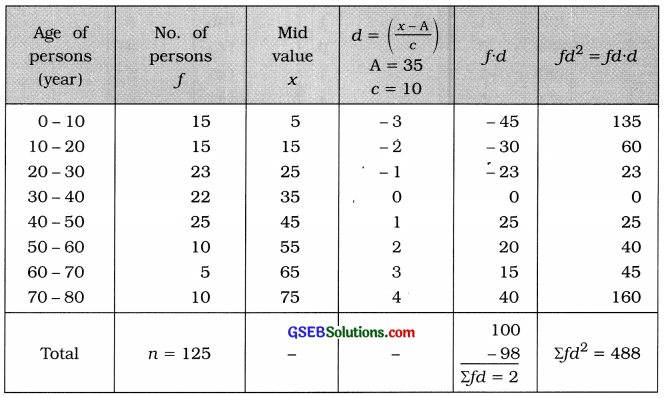x̄ = A + $$\frac{\Sigma f d}{n}$$ × c
= 35 + $$\frac{2}{125}$$ × 100
= 35 + $$\frac{20}{125}$$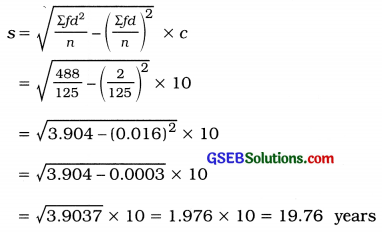= $$\frac{s}{\bar{x}}=\frac{19.76}{35.16}$$ = 0.56